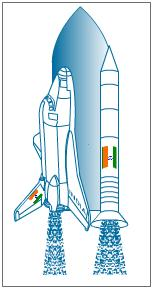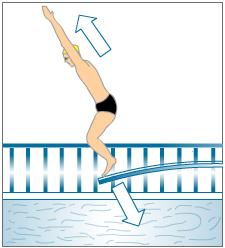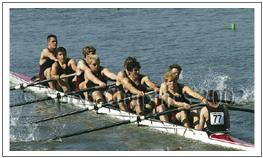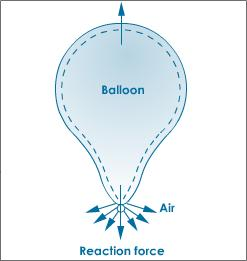• Have any questions?
• +91-9540-660064
• hahassignment@gmail.com

# Online Newtons Laws Of Motion Assignment Help

Newton's laws of motion consist of three physical laws that form the basis for classical mechanics. They describe the relationship between the forces acting on a body and its motion due to those forces. They have been expressed in several different ways over nearly three centuries, and can be summarized as follows:

First law: Every body remains in a state of rest or uniform motion (constant velocity) unless it is acted upon by an external unbalanced force. This means that in the absence of a non-zero net force, the center of mass of a body either remains at rest, or moves at a constant speed in a straight line.

Second law: A body of mass m subject to a net force F undergoes an acceleration a that has the same direction as the force and a magnitude that is directly proportional to the force and inversely proportional to the mass, i.e., F = ma. Alternatively, the total force applied on a body is equal to the time derivative of linear momentum of the body.

Third law: The mutual forces of action and reaction between two bodies are equal, opposite and collinear. This means that whenever a first body exerts a force F on a second body, the second body exerts a force −F on the first body. F and −F are equal in magnitude and opposite in direction. This law is sometimes referred to as the action-reaction law, with F called the "action" and −F the "reaction". The action and the reaction are simultaneous.

Introduction to newtons first law of motion:

Newton’s laws give a precise definition of force and a relationship between the force applied on a body and the state of motion acquired by it. Newton’s first law of motion describes the behaviour of bodies or objects around us, which are in a state of rest or in motion in a straight line.

### Newton’s first Law of Motion:

Newton’s first law of motion:

The first law of Newton’s states that “ A body continues in its state of rest or of uniform motion in a straight line unless an external force acts on it”. It means a body at test will remain at rest and a body in motion will remain in motion unless the body is compelled by some external force to change the state of rest or of uniform motion.

Newton’s first law of motion is also called as Galileo’s law of inertia. This law states that every body has some inertia.

Inertia:

Every body has a tendency to oppose any change in its state of rest or uniform motion in a straight line which is called inertia.

The meaning of inertia is unchanging. Thus not in inertia is the inherent property of the body by virtue of which it opposes any change in its state of rest or uniform motion in a straight line.

### Newtons Law of Motion with Types of Inertia and Examples:

Types of inertia:

• Inertia of rest
• Inertia of motion

Inertia of rest: Due to this inertia, a body at rest tends to remain at rest.

Inertia of motion: Due to this inertia, a body in uniform motion in a straight line tends to continue its motion, remaining unchanged.

Mass: The measure of inertia is mass. The larger the mass the larger in inertia. The smaller mass is the smaller inertia.

Examples of Newton’s first law of motion:

Examples of inertia of rest:

• When a bus starts suddenly, the passengers fall backwards
• Removal of dust from carpet.

Examples of inertia of motion:

• When a running bus stops suddenly, the passengers are jerked forward
• The passengers getting out of a moving train or bus falls in the forward direction

### Newton’s third Law of Motion:

Suppose you are watching the lift off of a space-shuttle. You hear a deafening roar and see burning gases shooting from the exhaust vents of the rockets. At that moment, the space shuttle system moves slowly upward. You can infer that the force for the lift off comes from the burning gases pushing against the shuttle rockets. Why does the shuttle system move in the opposite direction of the gases?

###Look carefully at the photo. Where is the interaction of forces the greatest in the rockets?

### Equal and Opposite Force in Newton's third law

The forces on the space-shuttle are similar to the forces in a collision between two tennis balls. When the balls collide, they are propelled in opposite directions. The rockets of the space-shuttle force burning gases downward through the exhaust vents. In response to these downward forces, the shuttle system moves upward. The motion of the space-shuttle demonstrates Newton's third law of motion.

When one object exerts a force upon a second object, the second object exerts an equal and opposite force upon the first object.

The third law of motion states that every action has an equal and opposite reaction. You can see equal and opposite forces interact when you blow up a balloon and release it, it moves in the opposite direction. The force propelling the balloon is equal and opposite to the force of the air leaving the balloon.

### Description of Balanced and Unbalanced Forces

Notice when the diver jumps down on a diving board. The board springs back and forces the diver into the air. The action force exerted on the board by the diver causes a reaction force by the board equal and opposite to the force exerted by the diving board.

###These interacting forces are unbalanced and differ from the balanced forces you studied in Newton's first law. Recall that no motion occurs when the forces are balanced. If the diver stands quietly on the diving board, all the forces on the diver and the board are balanced. Neither the diver not the board moves.

### Observing Newton's Third Law

The crew team uses Newton's third law of motion to move its boat. When an oar is put into the water, the water exerts an equal force on both sides of the oars, the surface of the flat side of the oars pushes against the water. The water pushes back on the oars with an equal and opposite force. The boat moves in the opposite direction of the oars with a force that is equal to that of the oars as they push against the water. The boat moves because the forces against it are unbalanced.

###Newton's third law of motion deals with action and reaction. To understand it, do the following experiments.

### Experiments to prove Newton's third law of motion

Hook two spring balances as shown in the diagram and pull from either side. You will notice that the pointers on the spring balances read equally (say 100 N). This shows that both the spring balances are registering equal force. Blow a balloon and hold its neck tightly facing downward. When you release the balloon, you will see that the balloon moves up instead of falling to the ground.

###Both the above experiments show that when a body exerts force on another body, the second body too exerts an equal and opposite force on the first body. The two forces are called action and reaction. The relation between an action and its reaction was given by Newton in the form of Newton's third law of motion. According to it, To every action there is an equal and opposite reaction In the first experiment, when two spring balances are pulled in opposite directions, they experience the same force acting in the opposite directions. In case of the balloon when you release it, the air inside the balloon rushes out downward, exerting force on the air outside. The balloon experiences equal and opposite reaction. Therefore, the balloon is pushed upward.

Rockets work on the same principle. The exhaust gases produced as the result of the combustion of the fuel are forced out at one end of the rocket. As a reaction, the main rocket moves in the opposite direction. It is important to note that action and reaction act on different bodies; action acts on one body and reaction on the other. Both begin and end at the same time. Some of the practical applications of Newton's third law of motion is illustrated in the diagram.

###In case you face any problem or have any query please email us at :-info@homeworkassignmenthelp.com

### Submit us an Assignment:

Our tutors start working only after the payment is made, to ensure that we are doing work only for serious clients and also our solution meets the required standard.

### Getting homework help was never so easy you just need to follow following steps:

• Send us you Other Assignment or problem through email
• Specify the required format such as Word, Excel, Notepad, PDF
• Give us a deadline when you need the assignment completed along with the Time Zone.
(for example: EST, Australian GMT etc)
• Send documents related to your assignment which can help our tutors to provide a better work,
any example or format you want the solutions to be in.
• Our tutors will review the assignment sent by you and if all the required information is there we will
send you the price quoted by our tutor along with the time needed to solve the assignment
• You can pay us through paypal or credit card.
• After receiving the payment tutors start working on your assignment.
• Finally, we deliver the solutions and get a feedback from you regarding our work

In case you face any problem or have any query please email us at :- info@homeworkassignmenthelp.com